If you are a "full stack" developer and don't understand database performance tuning, then you should learn. There isn't too much for any half-decent developer to learn but it is also easy to ignore performance until it is dreadful, even if doing so pushes you very close to performance problems with some really small queries.

For example, I worked somewhere where an address lookup by customer id took nearly 30 seconds! This sounded terrible (and it was) so I had a look at the query, which was written by some expensive DB consultants, and refactored it, so it now took less than half a second. So the difference can be astronomical between the same query written well and written badly.

### Index Basics

Many devs have heard of indexes and some even mention them when asked about database performance but even here, people start to struggle. This article isn't about indexes, but it helps to understand basically that a (non-clustered) index is a subset of the columns in a table which can make the WHERE part of the query use the, usually, much smaller index to find something that can avoid looking through every row in the table.

For example, imagine we were doing:

SELECT q.QuestionText from dbo.questions q where q.QuestionId = 12345

Since, in most cases, you would have a clustered index and very often it is on the Id column (primary key), this query would be able to dive into the clustered index very quickly (since it is ordered by QuestionId), just like looking for a surname in a phone book, we don't need to go through the entire book but can dive into the rough area we need and quickly find what we need (SQL Server is better than that but you get the idea). The result, an INDEX SEEK, is very fast because it avoids large scans and locking pages while looking for data.

Imagine instead, we ran this (without any indexes on the table other than the clustered PK index):

SELECT q.QuestionText from dbo.questions q where q.SurveyId = 12345

SQL Server has no way of knowing which rows in the table might have this survey id, so it will need to scan the entire table, looking for results. This results in a CLUSTERED INDEX SCAN which is really a TABLE SCAN and is going to be slow if your table is large.

What happens if we add a simple non-clustered index on SurveyId to the table and run the query above?

Answer, it depends. You are either going to see another CLUSTERED INDEX SCAN, if the table is small, or more likely, an INDEX SEEK (for the surveyid) followed by a KEY LOOKUP, which means we need to link our indexes results to the main index and lookup the additional column(s) we need (QuestionText in our case)

### Query Plans and Joins

OK, so if you are this far, you probably know something and hopefully have some experience of query execution plans. They look like this: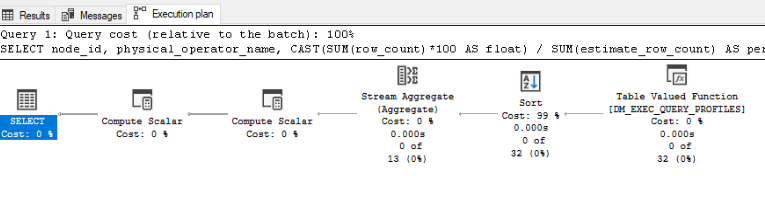Any time you need to improve query performance, this is very commonly where you start, you enable "Include Actual Execution Plan", run the query in SSMS and click on the Execution Plan tab. This example doesn't show anything useful because it is really very fast but you should be able to see that Sort was 99% of the cost of the query.

Now if this query took 2 minutes to run and Sort was taking 99%, then we would need to do something, ideally, to remove the sort! Remember that we could possibly perform sorting in client code or perhaps approach the problem another way. Sometimes we can completely butcher a query, perhaps using temporary tables or whatever to make something perform better but perhaps we have done this, added lots of indexes and now we are getting 100% INDEX SEEKs but still we are seeing problems, like the following plan: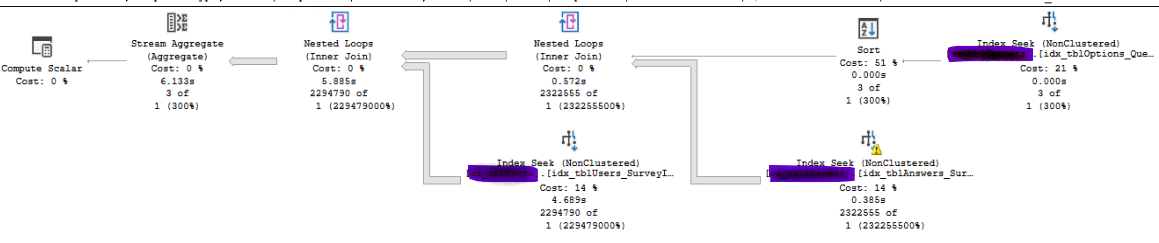We have three joined tables and they are all parsed using INDEX SEEK, which is great right? Well it is, but the query is still taking 9 seconds, which doesn't seem amazing, when the query is simply to group by a couple of columns and return 3 rows!

We have a large Sort problem again (51% of the 9 seconds) which is largely unavoidable because the groupby effectively has to order the results to group them and the index we are hitting is not ordered by  those columns.

### Statistics

However, something is happening which will bring us back to statistics. You might have noticed that sometimes an INNER JOIN shows up as Nested Loops and sometimes as Hash Match. They are different and a Hash match is generally faster on larger data sets. Well, we have a very large set of data, so why are we seeing a NESTED LOOP and not a HASH MATCH? There is a clue in the diagram: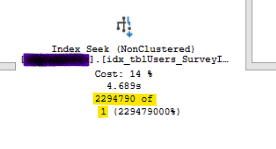What is 2294790 of 1? It means that the query thought that it would only get roughly 1 row in this query which means a nested loop will be faster. When the query actually ran, however, it got 2.3M rows instead so we suffered because of the query execution plan.

The good next question is why did it expect to only get 1 row back?

If you had a number of different ways of creating a query plan and they were based on the number of rows, how would you choose between them without having to scan entire tables? Well, we could create some basic statistics on the distribution of various columns through the table. For example, we could create statistics on the SurveyId column, which would describe that there are roughly 100 rows for each surveyid. These statistics are VERY basic, since anything complex would take up too much space and statistics can only take up to 8KB of space.

How do we create statistics? 3 ways.

1) You can create your own statistics and can optionally choose how much of the table to sample, to avoid hitting very large tables. If your data is less evenly distributed, you probably need a higher sample size or "fullscan"

2) You get statistics automatically for every index you create

3) You can enable "Auto Create Statistics" on the database and every time SQL finds a column in a query that does not have statistics, it creates one. This can lead to a lot of statistics of variable value and is generally only useful for simpler systems.

### How do they work?

If we show statistics, we can see a very basic histogram (in table form):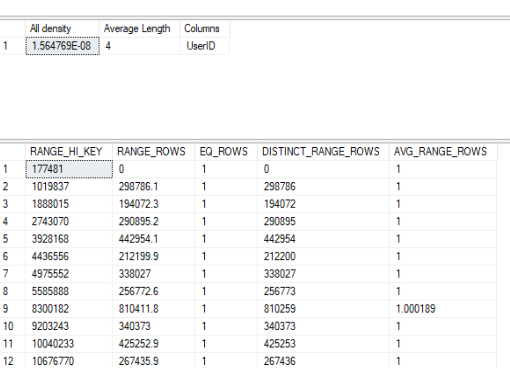You can see here the statistics for the primary key so it only covers UserID and each row in the bottom table says that for each range of primary keys, there is an average (unsurprisngly) of 1 row per userid. What is the effect of this? When I run a query WHERE UserId = X, it can deduce that there is likely to be roughly 1 row returned from this table and can optimise the query accordingly.

All good so far, but what happens if we have statistics on multiple columns? Well, as you can imagine, it cannot average all combinations of each of the columns so it only really covers the first column with an adjustment for each additional column: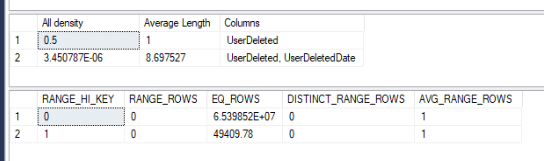So you can see here that 65M users are not deleted and 50K are deleted but when it comes to the Delete Date, there will be little useful data that we can determine since the dates will be all over the place but you can see that only a small percentage of all records have a UserDeletedDate.

So if this statistics was used for a query WHERE UserDeleted = 0, it would be very useful and would assume there will be 65M rows returned from the result. If we had WHERE UserDeleted = 0 AND UserDeletedDate IS NOT NULL, it would assume that 3.4*10-6 of the 65M rows would not be null. Note that in our case, it is very likely that only rows were UserDeleted = 1 would have a deleted date set but the statistics has no way of knowing this and will provide a wrong estimate for the number of rows (although probably in the correct order of magnitude in this instance).

### Improving Statistics

This is where is gets complicated. We already know that multiple column statistics only have partial value compared to single column where clauses. You should also know that the logic of which statistics to use if multiple ones overlap is not documented, newer ones? Ones that cover better? No way of knowing really.

So the first step is to find areas of your execution plan have very different estimates and actual, like our example above (2.3M of 1!) and we simply have to trace through how the query would use statistics to assume the number it has determined. In our case, if we hover over the box on the query plan, we can see the estimate is EXACTLY 1, not roughly 1. This might be a useful clue as to how it has determined it.

I have then removed all of the system statistics on the table and run the query again to make sure it is only seeing index statistics. I have then updated them with fullscan to get them as accurate as possible. If we can get them working well we might see if there is a problem with sampling that means we need to always update these table statistics with a full scan.

If I pick 1 of my tables and look at the seek predicates, I can see surveyid, questionid and optionid. I also know from the query that the first two are used in the where clause and the third one is used in a join. I do NOT expect it to guess anywhere near as high as 2M since this is an example of a large dataset but I would expect an average of perhaps a few thousand at least. The estimate should be based on the where clause, it cannot easily pre-empt the join results so I want to ask, "How would SQL Server approximate the number of rows with a fixed questionid and surveyid". I should also verify whether my assumption is correct about the average number of answers per-question-survey is correct. If the average is actually really low, I cannot expect SQL Server simply to know that this survey is large.

So after a rebuild of the statistics and running the query again, it is still wrong so now we need to consider how the statistics might work. Since we are using surveyid and questionid, we can quickly find a statistics that covers both of these: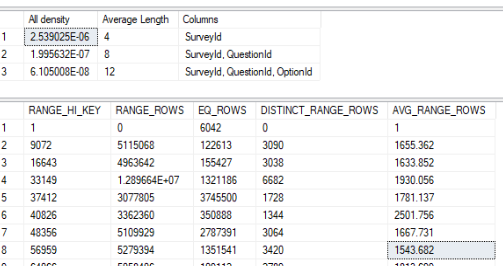As you can see here, the row (highlighted at the bottom) says that the surveyid I am looking for falls within a range that has an average of 1550 rows (answers) per surveyid, this would sound about correct, even though this actual survey has 2.5M (just proves that many surveys don't have any answers or certainly not many).

The problem comes here when you then consider the entry in the top table for SurveyId, QuestionId. you will see that it is saying that for every survey, there is, on average 0.0002 questions for each of these surveys. If we multiply 1543 by that number, we get about 0.3 rows so SQL Server is assuming for a specific surveyid and questionid, we would get 0.3 i.e. 1 row back. This is a major problem with statistics and the fact that questionids belong to surveys so there is not an even distribution of questions per survey. Effectively, specifying a question id does not reduce by as much as 5000 times but if a survey has 20 questions, specifying 1 would likely reduce the number of rows by only 20. Again, SQL Server cannot know this with generic data so the next question is how can we resolve this?

Having the index on the table is critical to getting query performance and provides a much better performance gain than having the best statistics setup but here the index has caused a problem because our data simply does not fit the averaging of the data distribution in the statistics and you cannot create the index without the statistics.

### Conclusion

So what can we do in our example? Possibly nothing here but otherwise we need to carefully consider the existing indexes (and whether they could be modified or dropped) whether we need new indexes, even overlapping indexes, so that a more specific index might provide more appropriate statistics for the joins.

If this is not possible, we would need to consider some other options, which are not necessarily better for all scenarios but might be:

1. Using temporary tables to put an exact subset of data in before joining it to your other tables, this way, you can create the exact indexes you need for sorting etc.
2. Batch the process so that it might be e.g. updating a table in the background for performance reasons and then getting data that is up to X minutes stale in return for the performance gain
3. Caching outside of the database so that only a percentage of calls are slow and the rest are instant, you might use this in conjunction with background refresh
4. Breaking up your data into smaller chunks to make it easier to handle. For example, some people are prone to treating entire lists as immutable instead of being able to invalidate/update only items within the list, especially if data in the tables is write-once.
5. Moving large data into other database systems e.g. ElasticSearch both to remove the query load from the production database but also to provide better performing queries.